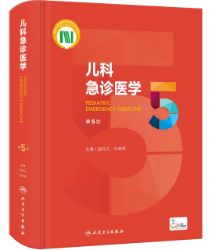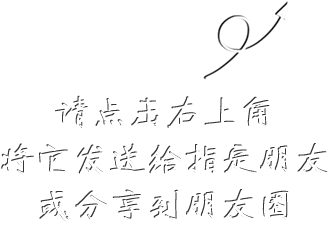998# 儿科急诊医学（第5版） 本书为预售，预计2022年6月30日后发货

## ¥ 158.40198.00现货已售出1件

1. 本书是一部大型儿科急诊医学专著，由国内一百余位急诊医学、重症医学和有关学科的专家撰写。内容丰富，资料新颖，科学性、系统性、实用性强，是儿科急诊医学科、重症医学科等相关学科人员等案头必备参考书。

2. 传承经典，本书内容已经更新至第5版，更注重临床实用性，突出儿科急诊、急救的专业特点，在原有的基础上补充近5年来的新进展、新的治疗方法，并删除已过时的内容，力求全面、实用、易于掌握。

3. 本版新增配套增值服务，视频内容实用、全面，更易于读者学习掌握。

• 删除
• 删除
• 删除
• 删除
• 删除
• 删除
• 删除
• 删除
• 删除
• 删除
• 删除
• 删除
• 删除
• 删除
• 删除
• 删除
• 删除
• 删除
• 删除
• 删除
• 删除
• 删除
• 删除
• 删除
• 删除
• 删除
• 删除
• 删除
• 删除
• 删除
• 删除
• 删除
• 删除
• 删除
• 删除
• 删除
• 删除
• 删除
• 删除
• 删除
• 删除
• 删除
• 删除
• 删除
• 删除
• 删除
• 删除
• 删除
• 删除
• 删除
• 删除
• 删除
• 删除
• 删除
• 删除
• 删除
• 删除
• 删除
• 删除
• 删除
• 删除
• 删除
• 删除
• 删除
• 删除
• 删除
• 删除
• 删除
• 删除
• 删除
• 删除
• 删除
• 删除
• 删除
• 删除
• 删除
• 删除
• 删除
• 删除
• 删除
• 删除
• 删除
• 删除
• 删除
• 删除
• 删除
• 删除
• 删除
• 删除
• 删除
• 删除
• 删除
• 删除
• 删除
• 删除
• 删除
• 删除
• 删除
• 删除
• 删除
• 删除
• 删除
• 删除
• 删除
• 删除
• 删除
• 删除
• 删除
• 删除
• 删除
• 删除
• 删除
• 删除
• 删除
• 删除
• 删除
• 删除
• 删除
• 删除
• 删除
• 删除
• 删除
• 删除
• 删除
• 删除
• 删除
• 删除
• 删除
• 删除
• 删除
• 删除
• 删除
• 删除
• 删除
• 删除
• 删除
• 删除
• 删除
• 删除
• 删除
• 删除
• 删除
• 删除
• 删除
• 删除
• 删除
• 删除
• 删除
• 删除
• 删除
• 删除
• 删除
• 删除
• 删除
• 删除
• 删除
• 删除